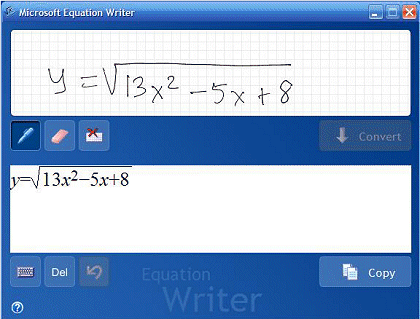Search IntMath
Close

# Equation Writer - at last!

By Murray Bourne, 19 Aug 2005

Update (Jun 2013): See my reviews of related products, MS Math 3.0 and MS Math 4.0.

Finally a math handwriting recognition software!

For years I have been wondering why it is that you can input Chinese characters using a digital pen and it converts it to 'nice' electronic characters, but you can't do the same with math?

Microsoft's Equation Writer, which comes with the Education Pack on a Tablet PC, does exactly what I have been wanting. This has a lot of potential in e-learning.Now, to buy a tablet PC to drive it...

Be the first to comment below.

### Comment Preview

HTML: You can use simple tags like <b>, <a href="...">, etc.

To enter math, you can can either:

1. Use simple calculator-like input in the following format (surround your math in backticks, or qq on tablet or phone):
a^2 = sqrt(b^2 + c^2)
(See more on ASCIIMath syntax); or
2. Use simple LaTeX in the following format. Surround your math with $$ and $$.
$$\int g dx = \sqrt{\frac{a}{b}}$$
(This is standard simple LaTeX.)

NOTE: You can mix both types of math entry in your comment.